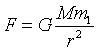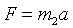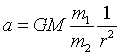QUESTION #6

# Do falling objects drop at the same rate (for instance a pen and a bowling ball dropped from the same height) or do they drop at different rates? I know a feather floats down very slowly but I would think a heavy object would fall faster than a light object. Thanks for your help. I have a bet on this one.

If no air resistance is present, the rate of descent depends only on how far the object has fallen, no matter how heavy the object is. This means that two objects will reach the ground at the same time if they are dropped simultaneously from the same height. This statement follows from the law of conservation of energy and has been demonstrated experimentally by dropping a feather and a lead ball in an airless tube.

When air resistance plays a role, the shape of the object becomes important. In air, a feather and a ball do not fall at the same rate. In the case of a pen and a bowling ball air resistance is small compared to the force a gravity that pulls them to the ground. Therefore, if you drop a pen and a bowling ball you could probably not tell which of the two reached the ground first unless you dropped them from a very very high tower.
Answered by: Dr. Michael Ewart, Researcher at the University of Southern California

The above answer is perfectly correct, but, this is a question that confuses many people, and they are hardly satisfied by us self-assured physcists' answers. There is one good explanation which makes everybody content -- which does not belong to me, but to some famous scientist but I can't remember whom (Galileo?); and I think it would be good to have it up here.

(The argument has nothing to do with air resistance, it is assumed to be absent. The answer by Dr. Michael Ewart answers that part already.)

The argument goes as follows: Assume we have a 10kg ball and a 1kg ball. Let us assume the 10kg ball falls faster than the 1kg ball, since it is heavier. Now, lets tie the two balls together. What will happen then? Will the combined object fall slower, since the 1kg ball will hold back the 10kg ball? Or will the combination fall faster, since it is now an 11kg object? Since both can't happen, the only possibility is that they were falling at the same rate in the first place.

Sounds extremely convincing. But, I think there is a slight fallacy in the argument. It mentions nothing about the nature of the force involved, so it looks like it should work with any kind of force! However, it is not quite true. If we lived on a world where the 'falling' was due to electrical forces, and objects had masses and permanent charges, things would be different. Things with zero charge would not fall no matter what their mass is. In fact, the falling rate would be proportional to q/m, where q is the charge and m is the mass. When you tie two objects, 1 and 2, with charges q1, q2, and m1, m2, the combined object will fall at a rate (q1+q2)/(m1+m2). Assuming q1/m1 < q2/m2, or object 2 falls faster than object one, the combined object will fall at an intermediate rate (this can be shown easily). But, there is another point. The 'weight' of an object is the force acting on it. That is just proportional to q, the charge. Since what matters for the falling rate is q/m, the weight will have no definite relation to rate of fall. In fact, you could have a zero-mass object with charge q, which will fall infinitely fast, or an infinite-mass object with charge q, which will not fall at all, but they will 'weigh' the same! So, in fact, the original argument should be reduced to the following statement, which is more accurate:

If all objects which have equal weight fall at the same rate, then _all_ objects will fall at the same rate, regardless of their weight.

In mathematical terms, this is equivalent to saying that if q1=q2 then m1=m2 or, q/m is the same for all objects, they will all fall at the same rate! All in all, this is pretty hollow an argument.

Going back to the case of gravity.. The gravitational force is( G is a constant, called constant of gravitation, M is the mass of the attracting body (here, earth), and m1 is the 'gravitational mass' of the object.)

And newton's law of motion iswhere m2 is the 'inertial mass' of the object, and a is the acceleration.

Now, solving for acceleration, we find:Which is proportional to m1/m2, i.e. the gravitational mass divided by the inertial mass. This is our old 'q/m' from the electrical case! Now, if and only if m1/m2 is a constant for all objects, (this constant can be absorbed into G, so the question can be reduced to m1=m2 for all objects) they will all fall at the same rate. If this ratio varies, then we will have no definite relation between rate of fall, and weight.

So, all in all, we are back to square one. Which is just canceling the masses in the equations, thus showing that they must fall at the same rate. The equality of the two masses is a necessity for general relativity, and enters it naturally. Also, the two masses have been found to be equal to extremely good precision experimentally. The correct answer to the question 'why objects with different masses fall at the same rate?' is, 'beacuse the gravitational and inertial masses are equal for all objects.'

Then, why does the argument sound so convincing? Since our daily experience and intuition dictates that things which weigh the same, fall at the same rate. Once we assume that, we have implicitly already assumed that the gravitational mass is equal to the inertial mass. (Wow, what things we do without noticing!). The rest of the argument follows easily and naturally...
Answered by: Yasar Safkan, Physics Ph.D. Candidate, M.I.T.